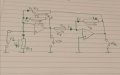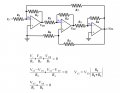# How do I calculate the voltage gain in a multistage opamp circuit if I dont know any voltages?

Joined Mar 4, 2017
5
Hey I just found this forum and was hoping someone here could help me. I really hope this is the right place for my question!My teacher gave me a practice exercise to get used to the idea of multistage amplifying circuits. I'm supposed to determine the voltage gain of the two stages.
R1=R2=R
R3=5R
R4=4R
R5=2R
The second stage seems rather straight forward to me (GV2=VO2/VO1).Because VO1=V4 and VO2=-V5 and it seems that I4=I5, the gain for that stage would simply be GV2=((-I4*2R)/4R*I4)=-2R/4R=-0.5

The second stage is proving to be more complicated though. I know this circuit is a non-inverting amplifier and that I should use the same approach (GV1=VO1/Vi). The resistor relationship is really confusing me though. I tried doing it as ((R1+R2||(R3+R4+R5))/((R2||(R3+R4+R5)) but the R3+R4+R5 part seems off..Thank you so much in advance for any responses xx

Lucia

#### WBahn

Joined Mar 31, 2012
25,597
Look at it in terms of the currents.

What is the current, flowing upward, in R1, in terms of Vi?

Can you express I2 and I3 in terms of Vi, V01, and V02?

Joined Mar 4, 2017
5
Heythank you! So I would say that because the circuit involves ideal op amps the voltage drop over R1 should be the input voltage? Then I1 would be V1/R1 so Vi/R1?

I2 is V2/R2...V2 would then be the difference between Vi and VO1?

Same for I3 except that the voltage drop would be the difference between Vi and VO2?

Am I going in the right direcction?

Thank you xx

#### WBahn

Joined Mar 31, 2012
25,597
Yes, you are going just fine. I had asked for the current going upward in R1, but by the passive sign convention what you have is just fine. Do note, however, that it is the current flowing downward in R1.

So how are I1, I2, and I3 related?

Joined Mar 4, 2017
5
Well, if we consider I1 to be going upwards.. then looking at the nodes that would mean that I1=I2+I3?

#### WBahn

Joined Mar 31, 2012
25,597
Well, if we consider I1 to be going upwards.. then looking at the nodes that would mean that I1=I2+I3?
Exactly. That's why I recommended defining I1 to be going upwards -- so that it would be equal to the sum of your other two currents. If I were doing the problem from scratch, I would probably have defined I1 to go down, since that would be the direction it would flow for positive input signal, but then defined both I2 and I3 to be going in the other direction so that, again, I1 would be the sum of I2 and I3.

At the end of the day you could flip a coin to determine the defined current directions -- as long as your equations are consistent with those choices. You choices are perfectly valid, you just have to be sure that you have

-I1 = I2 + I3

Joined Mar 4, 2017
5
Sorry if I'm being slow..but how do I go from here? I know I need to figure out Vi. It should be the same for both inputs and I should probably make use of the currents we just looked at yet somehow I can't figure out how to proceed from here

#### WBahn

Joined Mar 31, 2012
25,597
Sorry if I'm being slow..but how do I go from here? I know I need to figure out Vi. It should be the same for both inputs and I should probably make use of the currents we just looked at yet somehow I can't figure out how to proceed from here
"both inputs"? There's only one input -- Vi.

Can you find V02 in terms of V01? (I think you've already done this one)

Can you find V01 in terms of Vi and I2?

Can you find I2 in terms of I1 and I3?

You know what I1 is (in terms of Vi), right?

Can you find I3 in terms of Vi and Vo2?

Can you then find I2 in terms of Vi and Vo2?

You just need to piece these together until you make all of the voltages and currents except V02 and Vi go away.

Take your best shot and post your best attempt however far you get. Then we can help you get a bit further in each iteration.

#### MrAl

Joined Jun 17, 2014
7,518
Sorry if I'm being slow..but how do I go from here? I know I need to figure out Vi. It should be the same for both inputs and I should probably make use of the currents we just looked at yet somehow I can't figure out how to proceed from here
Hi,

You can also use superposition which will probably make this circuit a cakewalk in the park. Treat the input Vin and output Vout as separate sources, judiciously.

As your problems get more and more complicated you'll start to find you need more general means to solve them. It takes too much time to try to figure out all the nuances in every little circuit while a general method can get you there right away. Nodal analysis is one such method.

Joined Mar 4, 2017
5
"both inputs"? There's only one input -- Vi.
you're right, I meant both input terminals (inverting and non-inverting).

Can you find V02 in terms of V01? (I think you've already done this one)

Seeing as the gain for the second stage is VO2/VO1=-0.5, VO2=-0.5VO1

Can you find V01 in terms of Vi and I2?
this one I got stuck on.

Can you find I2 in terms of I1 and I3?

I1=V1/R1=Vi/R1=Vi/R where R is the given value of the resistance
I2=V2/R2=Vi-VO1/R2=Vi-VO1/R
if I substitute in I1 for Vi/R that leaves me with I2=I1-VO1/R

Or using the node rule I know that I2=-I1-I3

You know what I1 is (in terms of Vi), right?

I1=Vi/R1?

Can you find I3 in terms of Vi and Vo2?

I3=Vi-Vo2/R3

Can you then find I2 in terms of Vi and Vo2?
I2=Vi-Vo1/R2

You just need to piece these together until you make all of the voltages and currents except V02 and Vi go away.

Take your best shot and post your best attempt however far you get. Then we can help you get a bit further in each iteration

So I took the current equation I had (I2=-I1-I3) and substituted in the voltages and resistances:

Vi-Vo1/R2=-Vi/R1 - Vi-Vo2/R3

substituting in the values of the resistances:
Vi-Vo1/R=-Vi/R - Vi-Vo2/5R
and then multiplying by 5R:
5Vi-5Vo1=-5Vi-5Vi-5Vo2
if I then simplify and replace Vo2 by -0.5Vo1:
15Vi=2.5Vo1

#### RBR1317

Joined Nov 13, 2010
546
It would seem that the voltage gain of the two stages should be:

G = (Vo2)/(Vi)

So what is that number?

#### MrAl

Joined Jun 17, 2014
7,518
Hey I just found this forum and was hoping someone here could help me. I really hope this is the right place for my question!My teacher gave me a practice exercise to get used to the idea of multistage amplifying circuits. I'm supposed to determine the voltage gain of the two stages.
R1=R2=R
R3=5R
R4=4R
R5=2R
The second stage seems rather straight forward to me (GV2=VO2/VO1).Because VO1=V4 and VO2=-V5 and it seems that I4=I5, the gain for that stage would simply be GV2=((-I4*2R)/4R*I4)=-2R/4R=-0.5

The second stage is proving to be more complicated though. I know this circuit is a non-inverting amplifier and that I should use the same approach (GV1=VO1/Vi). The resistor relationship is really confusing me though. I tried doing it as ((R1+R2||(R3+R4+R5))/((R2||(R3+R4+R5)) but the R3+R4+R5 part seems off..View attachment 121814

Thank you so much in advance for any responses xx

Lucia

Hello,

I just took another quick look and noticed that something does not seem right with this circuit.

Note that the output of the second stage feeds back into the input of the first stage, and that both stages invert. That means the circuit has positive feedback, which usually is not the way this kind of circuit is usually done, and it may not even work as is. Some circuits have positive feedback but this does not look like one that would want to have that because positive feedback is implemented for a good reason and this circuit does not seem to have a good reason for that.

Did anyone try to simulate this yet? It could lock up as the output saturates which of course means the gain calculation would be meaningless.

If we flip the leads of the second stage we get a circuit that works. R4 then goes to ground and the non inverting input of the second stage op amp becomes the input so the second stage no longer inverts.

This was deceiving looking at first because the input is fed to the non inverting input of the first op amp so it appears that it does not invert, but it does invert the feedback signal.

#### RBR1317

Joined Nov 13, 2010
546
How do I calculate the voltage gain in a multistage opamp circuit if I dont know any voltages?
In a situation like that your best option is to treat the unknown voltages as variables, and write the circuit equations in terms of those variables. The method for writing circuit equations with voltage variables is nodal analysis where the variables are node voltages. As an added benefit, no currents need to be identified nor current directions specified.

Next an example. Assuming a rampant familiarity with nodal analysis procedure, the objective of the example is to demonstrate the relative simplicity of the node equations for op-amp circuits. While the example circuit is constructed entirely with resistors, a real circuit such as an active filter would include capacitors; but the node equations would still be fairly simple.

For those not already familiar with node analysis, note that each term in the equation is of the form voltage÷resistance which is current, so the node equation is a statement of Kirchoff's Current Law at that node, only without explicitly identifying any currents. When considering the following example, imagine how you would solve it by identifying the branch currents versus using nodal analysis. Equations are written for nodes n1,n2,n3 but n1 & n3 are virtual grounds at zero volts and for simplicity I have neglected to carry the zeroes into the node equations. (Attached is a pdf of a Maple worksheet solving the node equations.)#### Attachments

• 65.4 KB Views: 6

#### MrAl

Joined Jun 17, 2014
7,518
Hi,

Yes, very good. I see that the author chose a ridiculous value for the gain of the second stage but that's acceptable for a scholastic exercise i think, but hokum for the practical circuit (2 stage op amp circuit for a gain of 1.222 ha ha).

What else is kind of funny is that there is no good reason for using positive feedback here as the very input goes right to the non inverting terminal of the op amp. Not too big of a deal i guess for just an exercise though.

So the idea was to create a gain of 2, then follow up with a gain of 1/2, then provide a little positive feedback just to mix things up a little